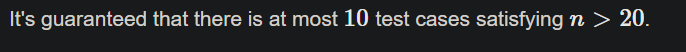# Target Time Complexity?

I’m looking at this problem from the Range DP module.

Is the target complexity \mathcal{O}(n^3)? I initially thought it would be \mathcal{O}(n^2), but then this part wouldn’t make sense:lol, being silver, I should probably not be helping you but if you want to check the editorial it says \mathcal{O}(n^3) (typos…)

Thanks!

1 Like